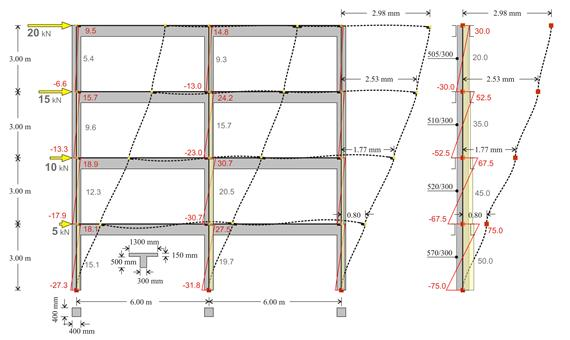# Deflection Of Beam In Structural Rigid Jointed Frame

This is a recommended follow on to one of my previous questions. It was stated that I could find the deflections of a beam by idealising it as a simply supported beam and then calculating accordingly. The loads laterally on the beam would cause a deflection perpendicular to the axis of the beam whereas the side-sway loads would cause deflections perpendicular to the column and thus the beam as well.

The main question is: how do I find the magnitude of the deflection on a particular beam within a rigid jointed multi-storey, multi-bay frame like the one shown below:How would I find the lateral deflection of each column and beam using traditional structural analysis methods? I can find the moments and all forces using moment distribution (Hardy Cross) method but I need the deflections in order to use serviceability limit states in design. I've also thought about the slope-deflection equation, however, I can only find the slopes at each end of the beams.

Also, how conservative are these results?

• Is it required that you use traditional methods? Direct Stiffness approach with static condensation seems like a fairly easy method for this problem. It does require inverting the stiffness matrix but you could use static condensation to make this simpler. – ShadowMan Oct 8 '19 at 20:34
• I prefer traditional methods mainly because most of the time, matrix methods (direct stiffness) requires something like excel to actually apply. I know there must exist an alternative traditional method because various structures were built even before the creation of software programs and computer programs. I mainly just need upper bounds of deflections. – Amit Oct 9 '19 at 1:53
• You can easily solve a matrix equation by hand. The alternative is to write out and solve the system of equations by hand, which is just more work to get the equivalent (remember, a stiffness matrix is nothing more than a set of equations). – Sanchises Oct 10 '19 at 10:36
• Yes, I could but I think it will most likely be faster to analyse individual beams using traditional methods rather than analysing the entire system and then having to check individual members later. These systems of equations that you mentioned, what are they? – Amit Oct 11 '19 at 10:57
• They are the compatibility and equilibrium equations in matrix form. i.e. direct stiffness method – ShadowMan Oct 11 '19 at 22:33